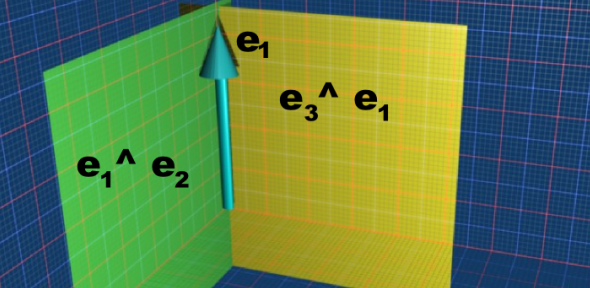# Maths - Clifford Algebra - Meet

We are looking for a similar operation to intersection in set theory or the 'AND' operation in logic. This operation would be very useful, especially when we extend these operations to elements that don't necessarily go through the origin, for instance we could use it to test for collision detection.

However such an operation would be non-linear and unstable. For example, if comparing two vectors, the only common element would be the origin (represented by a scalar), unless the vectors were exactly equal, when the result would be this common input vector. If one of these vectors moved very slightly then the result would suddenly switch back to a scalar, this would not be very practical for practical applications because rounding errors would cause unpredictable switching between the scalar and vector outputs.

### Meet Example

Lets start with the simple case of calculating the meet of two planes (in this example represented by the basis bivectors e3^e1 and e1^e2). The meet is e1 we can see that this is the vector common to both planes. In other words, the meet of two planes is the line where they cross.So how can we generalise this?

### Duality of Meet and Join

We can calculate the meet if we know the join or visa-versa. However the dual depends on the blade being considered, this means we can't get an expression for the complete multivector.

(AB)* = B* U A*

where:

• A,B are the two (different) bivectors.
•is the intersection (meet).
• U is the union (join).
• A* is the dual of A relative to the pseudoscalar of the subspace of the blade being considered.

Since the meet and join are both hard to calculate, this relationship does not necessarily help to calculate the meet of a given blade.

## Algorithm for calculating Meet

It seems to be very complicated to calculate the meet and join and I am struggling to understand it, I would welcome any help or ideas.

Here is an outline of the algorithm, note that it only combines two blades not whole multivectors. Let the two blades be A and B:

• If grade(A) > grade(B) swap A and B changing sign if necessary.
• calculate S=(A Δ B)* where:
• Δ = delta product
• * = dual for this subspace.
• factorise S to give si
• set M=1, J=In(In=is the pseudoscalar of the total space)
• for each si:
• pi = (si  A)  A-1
• ri = si-pi
• where pi is the projection of si and ri is the rejection of si
• If pi≠0 M=M^pi
• if grade(M)=reqM then calculate J from M and break
• otherwise continue with si+1
• If ri≠0 J=ri  J
• if grade(J)=reqJ then calculate M from J and break
• otherwise continue with si+1

An algorithm for calculating Meet and Join is given at Ian Bells website.

Also see 'Gaigen' a Geometric Algebra progam here.

Where I can, I have put links to Amazon for books that are relevant to the subject, click on the appropriate country flag to get more details of the book or to buy it from them.

This book has some explanation of an algorithm for calculating meet and join (section 21.7) but I don't claim to understand it .Geometric Algebra for Computer Science: An Object-oriented Approach to Geometry. This book stresses the Geometry in Geometric Algebra, although it is still very mathematically orientated. Programmers using this book will need to have a lot of mathematical knowledge. Its good to have a Geometric Algebra book aimed at computer scientists rather than physicists. There is more information about this book here.